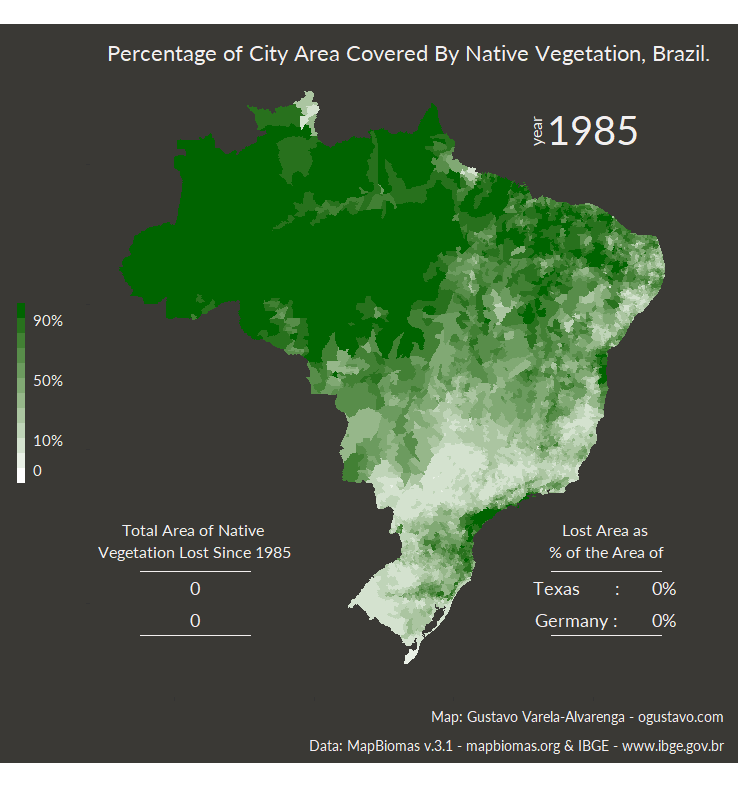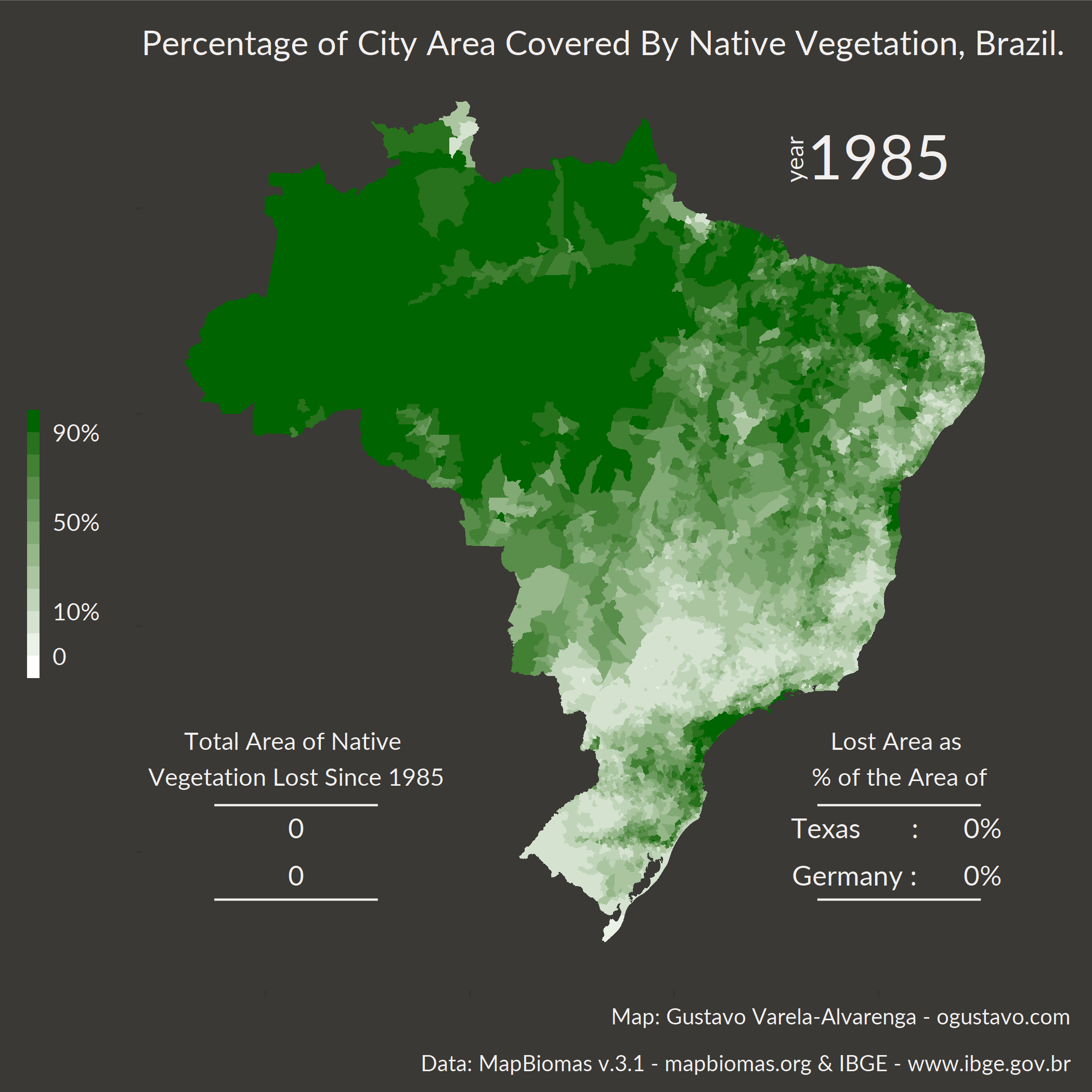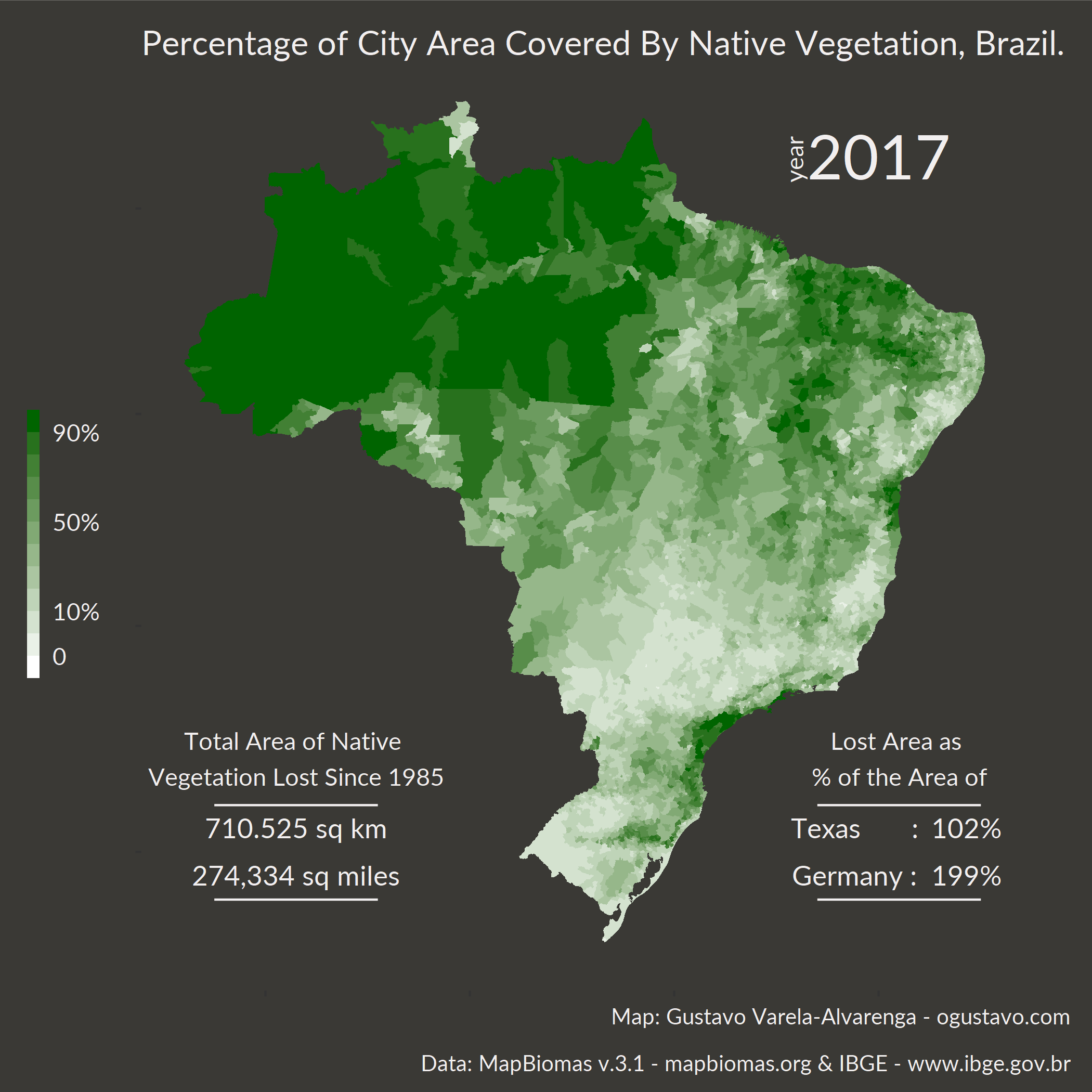# Create a Map in R with ggplot and transform it into a gif

## Section 1: Intro

In March 2019, the Brazilian Annual Land Use and Land Cover Mapping Project (MapBiomas) launched the Mapbiomas v.3.1 data set on land use and land cover for the period of 1985 to 2017.

This data set has information about the total area (in hectares) of forest formations, non-forest natural formations, farming, non-vegetated areas and water bodies - detailed information about these and their subdivisions can be found here.

And even though their website provides tools for anyone to create their own maps, I’ve decided to create my own animated map using R and the `ggplot2` and `gifski` packages because I wanted to see the percentage of a city area that is covered by native vegetation. I’m also using the `brazilmaps` package to plot Brazil’s map.

I wanted to focus on Natural Forest Formation data only and see how its total area evolved since 1985.

This tutorial has 3 sections, besides this introduction. The second section just goes over the packages and fonts that I used and how to download these fonts. In Section 3, I go over the data set and talk about its variables and their definitions. I also go over steps of importing the data set and doing some data manipulation to obtain the information that I want. Section 4 gives you the code for the final map.

You can download the `.R` file with the complete code from my GitHub.

## Section 2: Packages

I’ll begin by going over the fonts I used and how to install them. I use Windows, so I don’t know if the same steps apply to other systems (sorry Mac and Unix users).

I’m using Lato, an open-source font family. So, make sure you download and install the Lato font before we start or you can just let R use the default system font - you won’t have to change the code, you will get some warning messages, but R will still plot the map.

The first thing I do is make sure that R can use this font, so I’ll use the `extrafont` package for that. If it’s your first time using these fonts you’ll have to run the `font_import()` command (this might take a while depending on the number of installed fonts you have, the good thing is that you’ll have to run this command only once).

``````# ************************************************************************* ----
# extrafont Package                                                         ----
# ************************************************************************* ----

#install.packages("extrafont")

library("extrafont")

# ************************************************************************* ----
# Import Font                                                               ----
# ************************************************************************* ----

# Install font Lato

# Run font_import only once:

# font_import()

# Check if Lato was imported
fonts()[grep("Lato", fonts())]
``````
``````##  "Lato Black"    "Lato"          "Lato Hairline" "Lato Light"
##  "Lato Medium"   "Lato Thin"
``````
``````# Register the fonts with R

# ************************************************************************* ----
# Remaining Packages                                                        ----
# ************************************************************************* ----

#install.packages("openxlsx")
#install.packages("tidyverse")
#install.packages("brazilmaps")
#install.packages("gifski")

library("openxlsx")
library("tidyverse")
library("brazilmaps")
library("gifski")
``````

## Section 3: The Data

### Credit Where Credit is Due

The MapBiomas data are public, open and free through the simple reference of the source:

“Project MapBiomas - Collection 3.1 of Brazilian Land Cover & Use Map Series, accessed on 04/24/2019 through the link: (http://mapbiomas.org/pages/estatisticas")

“MapBiomas Project - is a multi-institutional initiative to generate annual land cover and use maps using automatic classification processes applied to satellite images. The complete description of the project can be found at http://mapbiomas.org

I’m also using data on the area of the cities in Brazil from IBGE (the Brazilian Institute of Geography and Statistics). Due to changes in the size of the cities over the years, I’ve decided to use data from 2016. I was getting some incorrect results using data from 2017 or 2018, like cities with over 100% of their area covered by native vegetation. Clearly, these cities were divided but this wasn’t accounted for in MapBiomas’ data set. No cities with over 100% of their area covered by native vegetation were observed when using 2016 data.

The code below imports the data sets to R, but if you want you can download the xlsx files from the official sites by clicking here for MapBiomas’ data set and here for IBGE’s data set.

For the sake of maintaining my code, I’m also uploading the original databases to my GitHub repository and that’s the data I’ll use in my code below.

### Data Dictionary

MapBiomas’ xlsx file has the following worksheet:

• INSTRUCTIONS;
• LAND COVER - BIOMAS e UF;
• LAND COVER - MUN_UF;
• CONSULTA BIOMA-UF; and
• CONSULTA MUN-UF.

I’ll use the LAND COVER - MUN_UF worksheet. The variables in this worksheet are:

Variable Class Description
CODIBGE numeric city identifier
municipio character city name
cod.classe numeric land cover class identifier
nivel1 character class level 1
nivel2 character class level 2
nivel3 character class level 3
1985 numeric covered area in hectares(ha) in 1985
2017 numeric covered area in hectares(ha) in 2017

IBGE’s xlsx file has the following worksheet.

• AR_BR_MUN_2016;
• AR_BR_UF_2016;
• AR_BR_RG_2016; and
• AR_BR_2016.

I’ll use the AR_BR_MUN_2016 worksheet. The variables in this worksheet are:

Variable Class Description
ID numeric ID
CD_GCUF character state identifier
NM_UF character state name
NM_UF_SIGLA character state abbreviation
CD_GCMUN character city identifier
NM_MUN_2016 character city name
AR_MUN_2016 numeric area in squared km

I’m also creating the data set `brazil.map` with the shapefiles to plot Brazil’s map divided by city using the function `brazilmaps::get_brmap`.

``````# ************************************************************************* ----
# Data Import                                                               ----
# ************************************************************************* ----
# Change number format
options(scipen=10)

# File Path on GitHub
file_path_forest_area = paste0(
"https://github.com/gu-stat/forest-area/blob/master/",
"?raw=true")

file_path_city_area = paste0(
"https://github.com/gu-stat/forest-area/blob/master/",
"data/AR_BR_RG_UF_MUN_2016.xlsx",
"?raw=true")

# Import
sheet = "LAND COVER - MUN_UF")

sheet = "AR_BR_MUN_2016")

## \__ Map Data by City ----

brazil.map <- get_brmap(geo = "City", class = "sf")
``````

### Data Manipulation

``````# ************************************************************************* ----
# Data Manipulation                                                         ----
# ************************************************************************* ----

## \__ Transform City Code into Numeric ----

df.city.area2 <-
df.city.area %>%
dplyr::mutate(CODIBGE = as.numeric(CD_GCMUN)) %>%
dplyr::select(CODIBGE, AR_MUN_2016)

## \__ Natural Forest Coverage Area Data ----

df.nat.forest <-
df.original %>%
filter(nivel2 == "Floresta Natural") %>%
paste0(seq(1985, 2017))
) %>%
# SUM THE DIFFERENT SUB-GROUPS OF NATURAL FOREST
summarize_at(vars(-nivel2,-nivel3),sum) %>%
ungroup()

## \__ Join with City Area Data ----

df.nat.forest.AREA <-
df.nat.forest %>%
# ADD TOTAL AREA OF MUNICIPALITY TO DATA SET
dplyr::left_join(x = ., y = df.city.area2, by = "CODIBGE") %>%
# CREATE PERCENTAGES
## Divide x/100 to transform from hectares to squared km
dplyr::mutate_at(.vars=vars(paste0(seq(1985,2017))),
.fun = function(x) round(100*((x/100)/.\$AR_MUN_2016),3)
)

# CHECK IF ANYTHING GREATER THAN 100%
greater.1 <- NULL
for (i in 1985:2017) {
greater.1 <- append(greater.1, which(df.nat.forest.AREA[,paste0(i)] >= 100))
}

greater.1 <- greater.1 %>% unlist() %>% unique()

## \__ Create Percentages Breaks ----

df.nat.forest.AREA <-
df.nat.forest.AREA %>%
dplyr::mutate_at(.vars=vars(paste0(seq(1985,2017))),
.fun = function(x) cut(x,breaks=c(-Inf, 0, 1, seq(0, 100, 10)[-1]))
)
``````

## Section 4: The Map

``````# ************************************************************************* ----
# Map                                                                       ----
# ************************************************************************* ----

## \__ Function that Joins Map Data by City with Forest Area Data ----

create.map.df <- function(DATA){

map <- join_data(map = brazil.map, data = DATA, by = c("City" = "CODIBGE"))

map.ggplot <- as(map, "Spatial")

map.ggplot@data\$id = row.names(map.ggplot@data)

df.map.ggplot <- suppressMessages(ggplot2::fortify(map.ggplot))

df.map.ggplot <- dplyr::left_join(df.map.ggplot, map.ggplot@data, by = "id")

return(df.map.ggplot)
}

## \____ Map Data for Plot ----

df.map.ggplot <- create.map.df(df.nat.forest.AREA)

## \__ Map Colors ----

# USE https://gka.github.io/palettes TO GET
# COLOR SCALE FROM white TO darkgreen
# THAT'S ALSO SUITABLE TO COLORBLIND PEOPLE WITH 12 BREAKS
# SAME NUMBER AS THE LEVELS OF cut(x,breaks=c(-Inf, 0, 1, seq(0, 100, 10)[-1]))

map.colors <- c('#ffffff','#eaf1e7','#d4e2cf','#bfd4b8','#abc5a1','#96b78a',
'#81a974','#6c9b5f','#588d4a','#428034','#28711d','#006400')

### \__ Map Labels ----

map.labels <- c("0", "", "10%", rep("",3), "50%", rep("",3), "90%", "")

## \__ Create Theme ----

map.theme <-
theme(
text = element_text(size = 13, family = "Lato", color = "#f2efef"),
axis.text = element_blank(),
axis.title.x = element_blank(),
axis.title.y = element_blank(),
plot.background = element_rect(fill = "#3a3935", color = NA),
panel.background = element_rect(fill = "#3a3935", color = NA),
panel.grid = element_blank(),
plot.margin = margin(t = 0.25,r = 0.25,b = 0.25,l = 0.25,unit = "cm"),
plot.title = element_text(margin = margin(t = 0.25, unit = "cm")),
plot.caption = element_text(size = 10),
legend.background = element_rect(fill = "#3a3935", color = "#3a3935"),
legend.text = element_text(size = 11),
legend.title = element_text(size = 12),
legend.position = "left",
legend.key = element_rect(color = "transparent"),
legend.key.size = unit(0, units = "mm"),
legend.key.width = unit(2, units = "mm")
)

## \__ Plot Map ----

### \____ Function for GIF ----
plot.map.forest <- function(){
for (i in 1985:2017) {

lost.area.year.km2 <-
round(
sum(-(df.nat.forest %>% summarize_at(paste0(i),sum)/100),
df.nat.forest %>% summarize_at(paste0(1985),sum)/100)
,2
)

lost.area.year.mi2 <- round(lost.area.year.km2/2.59, 2)

map.forest <-
# MAP
ggplot(mapping = aes(x = long, y = lat, group = group)) +
# MAP WITH TRANSPARENT CITY LINES
geom_polygon(
data = df.map.ggplot,
aes_string(fill = paste0("X",i)),
color = "transparent"
) +
# LEGEND
scale_fill_manual(
values = map.colors,
drop = FALSE,
na.value = "black",
na.translate = FALSE,
name = "",
label = map.labels,
guide = guide_legend(
direction = "vertical",
ncol = 1,
reverse = TRUE,
label.vjust = 2.5
)
) +
# MAP LIMITS
xlim(range(df.map.ggplot\$long)) +
ylim(range(df.map.ggplot\$lat)) +
coord_map() +
labs(
title = "Percentage of City Area Covered By Native Vegetation, Brazil.",
caption = paste0("Map: Gustavo Varela-Alvarenga - ogustavo.com \n \n ",
"Data: MapBiomas v.3.1 - mapbiomas.org & ",
"IBGE - www.ibge.gov.br ")
) +
map.theme +
## WORD YEAR
annotate(
geom = "text", x = -44.1, y = 2.4, size = 4, family = "Lato",
color = "#f2efef", label = "year", angle = 90
) +
## ACTUAL YEAR
annotate(
geom = "text", x = -40, y = 2.5, size = 10, family = "Lato",
color = "#f2efef", label = paste0(i)
) +
## TITLE - TABLE ON THE LEFT
annotate(
geom = "text", x = -68.5, y = -26, size = 4, family = "Lato",
color = "#f2efef", hjust = 0.5,
label =
paste0("Total Area of Native \n", "Vegetation Lost Since 1985")
) +
## LINE BELOW TITLE
annotate(
geom = "segment", x = -64.5, xend = -72.5, y = -28, yend = -28,
linetype = "solid", color = "#f2efef"
) +
## VALUES IN KM2 WITH . TO MARK THOUSANDS
annotate(
geom = "text", x = -68.5, y = -29, size = 4.5, family = "Lato",
color = "#f2efef", hjust = 0.5,
label =
ifelse(lost.area.year.km2 == 0, "0",
paste0(prettyNum(round(lost.area.year.km2, 0),
big.mark=".", decimal.mark = ","),
" sq km")
)
) +
## VALUES IN MI2 WITH , TO MARK THOUSANDS
annotate(
geom = "text", x = -68.5, y = -31, size = 4.5, family = "Lato",
color = "#f2efef", hjust = 0.5,
label =
ifelse(lost.area.year.mi2 == 0, "0",
paste0(prettyNum(round(lost.area.year.mi2, 0), big.mark=","),
" sq miles")
)
) +
## LINE BELOW TABLE
annotate(
geom = "segment", x = -64.5, xend = -72.5, y = -32, yend = -32,
linetype = "solid", color = "#f2efef"
) +
## TITLE - TABLE ON THE RIGHT
annotate(
geom = "text", x = -39, y = -26, size = 4, family = "Lato",
color = "#f2efef", hjust = 0.5,
label =  paste0("Lost Area as \n", "% of the Area of")
) +
## LINE BELOW TITLE
annotate(
geom = "segment", x = -35, xend = -43, y = -28, yend = -28,
linetype = "solid", color = "#f2efef"
) +
annotate(
geom = "text", x = -41, y = -29, size = 4.5, family = "Lato",
color = "#f2efef", label =  "Texas       : "
) +
annotate(
geom = "text", x = -34, y = -29, size = 4.5, family = "Lato",
color = "#f2efef", hjust = 1,
label =
ifelse(
lost.area.year.km2 == 0, "0%",
paste0(round(lost.area.year.km2/696241, 2) * 100, "%")
)
) +
annotate(
geom = "text", x = -41, y = -31, size = 4.5, family = "Lato",
color = "#f2efef", label =  "Germany : "
) +
annotate(
geom = "text", x = -34, y = -31, size = 4.5, family = "Lato",
color = "#f2efef", hjust = 1,
label =
ifelse(
lost.area.year.km2 == 0, "0%",
paste0(round(lost.area.year.km2/357386, 2) * 100, "%")
)
) +
## LINE BELOW TABLE
annotate(
geom = "segment", x = -35, xend = -43, y = -32, yend = -32,
linetype = "solid", color = "#f2efef"
)

print(map.forest)

}
}
``````
``````### \____ Create GIF ----
### Be mindful that this will save a gif file named forest_animation.gif into

gif_file <-
gifski::save_gif(
expr = plot.map.forest(),
gif_file = "forest_animation.gif",
delay = 0.75, width = 738, height = 788, res = 100
)

utils::browseURL(gif_file)
``````If you want you can you use the code below to get high-quality static images of the maps. Be mindful that this will save the plots into your working directory.

``````#install.packages("Cairo")
library("Cairo")

for (i in 1985:2017) {

lost.area.year.km2 <-
round(
sum(-(df.nat.forest %>% summarize_at(paste0(i),sum)/100),
df.nat.forest %>% summarize_at(paste0(1985),sum)/100)
,2
)

lost.area.year.mi2 <- round(lost.area.year.km2/2.59, 2)

map.forest <-
# MAP
ggplot(mapping = aes(x = long, y = lat, group = group)) +
# MAP WITH TRANSPARENT CITY LINES
geom_polygon(
data = df.map.ggplot,
aes_string(fill = paste0("X",i)),
color = "transparent"
) +
# LEGEND
scale_fill_manual(
values = map.colors,
drop = FALSE,
na.value = "black",
na.translate = FALSE,
name = "",
label = map.labels,
guide = guide_legend(
direction = "vertical",
ncol = 1,
reverse = TRUE,
label.vjust = 2.5
)
) +
# MAP LIMITS
xlim(range(df.map.ggplot\$long)) +
ylim(range(df.map.ggplot\$lat)) +
coord_map() +
labs(
title = "Percentage of City Area Covered By Native Vegetation, Brazil.",
caption = paste0("Map: Gustavo Varela-Alvarenga - ogustavo.com \n \n ",
"Data: MapBiomas v.3.1 - mapbiomas.org & ",
"IBGE - www.ibge.gov.br ")
) +
map.theme +
## WORD YEAR
annotate(
geom = "text", x = -44.1, y = 2.4, size = 4, family = "Lato",
color = "#f2efef", label = "year", angle = 90
) +
## ACTUAL YEAR
annotate(
geom = "text", x = -40, y = 2.5, size = 10, family = "Lato",
color = "#f2efef", label = paste0(i)
) +
## TITLE - TABLE ON THE LEFT
annotate(
geom = "text", x = -68.5, y = -26, size = 4, family = "Lato",
color = "#f2efef", hjust = 0.5,
label =
paste0("Total Area of Native \n", "Vegetation Lost Since 1985")
) +
## LINE BELOW TITLE
annotate(
geom = "segment", x = -64.5, xend = -72.5, y = -28, yend = -28,
linetype = "solid", color = "#f2efef"
) +
## VALUES IN KM2 WITH . TO MARK THOUSANDS
annotate(
geom = "text", x = -68.5, y = -29, size = 4.5, family = "Lato",
color = "#f2efef", hjust = 0.5,
label =
ifelse(lost.area.year.km2 == 0, "0",
paste0(prettyNum(round(lost.area.year.km2, 0),
big.mark=".", decimal.mark = ","),
" sq km")
)
) +
## VALUES IN MI2 WITH , TO MARK THOUSANDS
annotate(
geom = "text", x = -68.5, y = -31, size = 4.5, family = "Lato",
color = "#f2efef", hjust = 0.5,
label =
ifelse(lost.area.year.mi2 == 0, "0",
paste0(prettyNum(round(lost.area.year.mi2, 0), big.mark=","),
" sq miles")
)
) +
## LINE BELOW TABLE
annotate(
geom = "segment", x = -64.5, xend = -72.5, y = -32, yend = -32,
linetype = "solid", color = "#f2efef"
) +
## TITLE - TABLE ON THE RIGHT
annotate(
geom = "text", x = -39, y = -26, size = 4, family = "Lato",
color = "#f2efef", hjust = 0.5,
label =  paste0("Lost Area as \n", "% of the Area of")
) +
## LINE BELOW TITLE
annotate(
geom = "segment", x = -35, xend = -43, y = -28, yend = -28,
linetype = "solid", color = "#f2efef"
) +
annotate(
geom = "text", x = -41, y = -29, size = 4.5, family = "Lato",
color = "#f2efef", label =  "Texas       : "
) +
annotate(
geom = "text", x = -34, y = -29, size = 4.5, family = "Lato",
color = "#f2efef", hjust = 1,
label =
ifelse(
lost.area.year.km2 == 0, "0%",
paste0(round(lost.area.year.km2/696241, 2) * 100, "%")
)
) +
annotate(
geom = "text", x = -41, y = -31, size = 4.5, family = "Lato",
color = "#f2efef", label =  "Germany : "
) +
annotate(
geom = "text", x = -34, y = -31, size = 4.5, family = "Lato",
color = "#f2efef", hjust = 1,
label =
ifelse(
lost.area.year.km2 == 0, "0%",
paste0(round(lost.area.year.km2/357386, 2) * 100, "%")
)
) +
## LINE BELOW TABLE
annotate(
geom = "segment", x = -35, xend = -43, y = -32, yend = -32,
linetype = "solid", color = "#f2efef"
)

### Be mindful that this will save several png files named Y*.png,
### where * is the year, into your working directory

ggsave(
paste0("Y",i,".png"), device="png", type="cairo",
width = 7, height = 7
)

}
``````